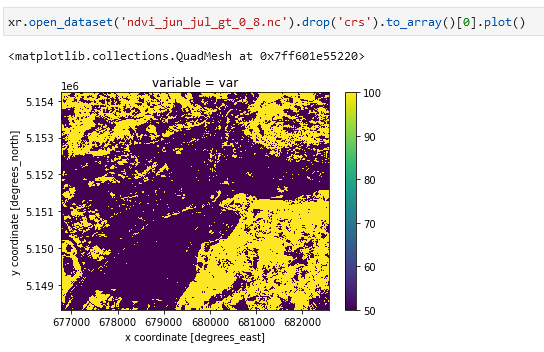# Conditional replacement e.g. where(condition, true, false)

Hi,

I have two datacubes and I want to evaluate where both are valid (not masked), if the condition is true I want to return one value, if false another.

What is the best way to do conditional replacement?

I’ve created an example for your use case.
In the example I compute the median NDVI for two months, then I check where the NDVI is greater than a value for both months and at the end I replace 0 and 1 with other values.

``````import openeo
from openeo.processes import eq, not_
import xarray as xr

openeoHost = 'https://openeo.cloud'
conn = openeo.connect(openeoHost).authenticate_oidc('egi')

'SENTINEL2_L2A_SENTINELHUB',
spatial_extent={'west': 11.304588, 'east': 11.377716, 'south': 46.465311, 'north': 46.516839},
temporal_extent=['2021-06-01', '2021-08-01'],
bands = ['B04','B08'],
properties={
'eo:cloud_cover': lambda x: lte(x, 50)
})
median_ndvi_june = im.filter_temporal(['2021-06-01', '2021-07-01']).ndvi().reduce_dimension(dimension='t',reducer='median')
median_ndvi_july = im.filter_temporal(['2021-07-01', '2021-08-01']).ndvi().reduce_dimension(dimension='t',reducer='median')

# Check weather the median NDVI is above 0.8 in both months. If yes set the area to 100, else to 50

value_0 = 100
value_1 = 50

# Firstly create a boolean mask for each month

# Merge the masks with a boolean AND operation

# Create the inverse of it

# Use the mask operator twice (with the mask and the inverse of it) to set two different values to replace 0 and 1

# Alternative approach using multiply + mask

``````

result:Thanks, looks close to what I want. However, what if i want the replacement value to be a datacube rather than a constant? In the documentation it doesn’t look like that’s possible.

You can do that, but not only with mask, you would need to combine it with merge_cubes.
Look at the modified example:

``````import openeo
from openeo.processes import eq, not_
import xarray as xr

openeoHost = 'https://openeo.cloud'
conn = openeo.connect(openeoHost).authenticate_oidc('egi')

'SENTINEL2_L2A_SENTINELHUB',
spatial_extent={'west': 11.304588, 'east': 11.377716, 'south': 46.465311, 'north': 46.516839},
temporal_extent=['2021-06-01', '2021-08-01'],
bands = ['B04','B08'],
properties={
'eo:cloud_cover': lambda x: lte(x, 50)
})
median_ndvi_june = im.filter_temporal(['2021-06-01', '2021-07-01']).ndvi().reduce_dimension(dimension='t',reducer='median')
median_ndvi_july = im.filter_temporal(['2021-07-01', '2021-08-01']).ndvi().reduce_dimension(dimension='t',reducer='median')

# Replace values of june NDVI with july NDVI when NDVI in June is > 0.8

# Firstly create a boolean mask for each month

# Set to zero the values in June where NDVI < 0.8
Thanks Michele! Your nice examples helped me get the solution I wanted